Aptitude Tests 4 Me

Non Verbal Logic

Detailed solution

121.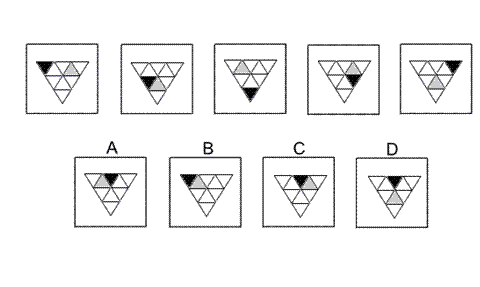Solution: A Explanation: In this question there is a big equilateral triangle divided up into a grid of nine equilateral triangles, one of which is shaded black and one shaded grey. The black and grey triangle each moves according to its own rule. The black triangle moves one place anticlockwise around the six small triangles that share an edge with the big triangle. Following this rule, in the next diagram of the sequence, the triangle in the centre at the top should be shaded black. The correct answer, therefore, could be A, C or D. The grey triangle moves one place clockwise around the three small triangles that do not share an edge with the big triangle. Following this rule, the grey triangle will move to the top left of the three. The correct answer, therefore, is A.

122.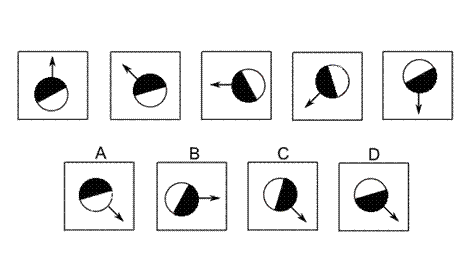Solution: D Explanation: In this question there are two rules that are performed simultaneously. The first rule is that the shape is reflected in the axis that follows the direction of the arrow. The second rule is that the shape is rotated by 45° anticlockwise each time. Following the second rule, the answer could be A, C or D. But the first rule tells us that the correct answer must be D. In A, the wrong half of the circle is coloured black. In C, the shape has not been reflected.

123.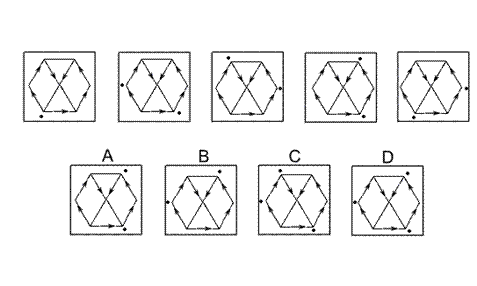Solution: D Explanation: In this question there is a network of arrows around five edges and two diagonals of a hexagon. The dot moves in the direction of the arrow to the next vertex of the hexagon. However, if there are two possible routes, the dot multiplies to two dots with one dot following each of the possible routes. Following this rule, the dot in the bottom left of the hexagon in the fifth diagram will multiply so that, in the next diagram of the sequence, there will be three dots altogether. The correct answer, therefore, is D.

124.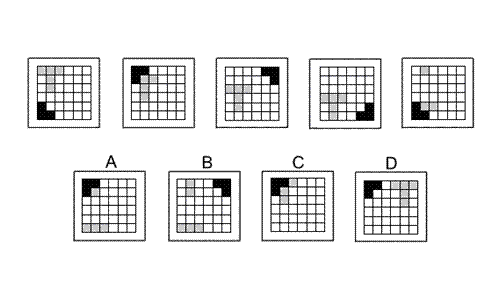Solution: A Explanation: In this question there is a grid of squares with a black L-shape and a grey coloured T-shape. The L-shape rotates around the corners of the grid by 90° clockwise each time. Following this rule, the correct answer could be A, C or D. The T-shape moves down one place each time. When the T-shape (or part of the T-shape) reaches the bottom row, it moves in the next diagram of the sequence to the top row. However, sometimes parts of the grey T-shape are obscured by the black L-shape. Following this rule, the correct answer must be A.

125.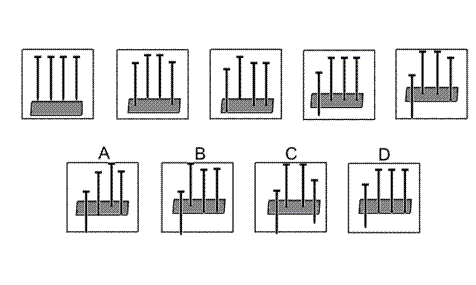Solution: B Explanation: In this question there is a block of wood and four nails. Each time the first nail (on the left) and one other nail are hit with a hammer - the first time, the first and fourth nails are hit, the second time the first and third nails are hit, the next time the first and second, then back to the first and fourth etc. For the next diagram of the sequence, therefore, the first and third nails should be hit. The correct answer, therefore, is B.

 1 2 3 4 5 6 7 8 9 10 11 12 13 14 15 16 17 18 19 20 21 22 23 24 25 26 27 28 29 30 31 32 33 34 35 36 37 38 39 40 41 42 43 44 45 46 47 48 49 50 51 52 53 54 55 56 57 58 59 60 61 62 63 64 65 66 67 68 69 70 71 72 73 74 75 76 77 78 79 80 81 82 83 84 85 86 87 88Passage ReadingVerbal LogicNon Verbal LogicNumerical LogicData InterpretationReasoningAnalytical AbilityBasic NumeracyAbout UsContactPrivacy PolicyMajor TestsFAQ